Categories

# Geostrophic Balance

We now turn to the forces driving horizontal motions in the atmosphere. Horizontal pressure gradients resulting from differential heating produce motions directed from high- to low-pressure areas. A complication is that the Earth is a rotating sphere, where different points have different translational velocities in a fixed frame of reference. The useful frame of reference for us is one that rotates with the sphere, since we measure all air motions with respect to this frame of reference. From the perspective of this rotating frame of reference, any motion taking place in the fixed frame of reference (such as driven by a pressure-gradient force) will be deflected due to the rotation. The deflection accelerates the air parcel away from its original direction and thus behaves as a fictitious force, called the Coriolis force. The Coriolis force operates in three dimensions but is negligible in the vertical relative to the acceleration of gravity. It is of critical importance for large-scale motions in the horizontal direction.To understand the Coriolis effect, consider that the translational Earth’s rotation velocity VT (directed eastward)

VT = a Ω cos φ (29)

decreases with increasing latitude φ. With an Earth’s radius a = 6378 km and an angular rotation velocity Ω = 7.292 × 10–5 rad s–1 (or 2 π rad d–1), the velocity VT is 1672 km h–1 at the Equator and 836 km h–1 at 60° latitude. If we consider an air parcel that is displaced poleward in the northern hemisphere, starting from latitude φ1, the conservation of angular momentum in the absence of external forces requires that the product ρa V(φ) a cos φ remain constant at its initial value ρa VT1a cos φ1 during the displacement of the parcel. Here, V is the absolute eastward velocity of the air parcel in the fixed frame of reference, and ρa is the air density. Since VT(φ) decreases with latitude φ, this condition can only be fulfilled if, for an observer located at the Earth’s surface, the air parcel acquires a gradually increasing eastward velocity. For the same reason, an air parcel moving toward the Equator in the northern hemisphere will be displaced westward (see Figure 8).

Figure 8 Trajectory of an object (such as an air parcel) directed from the north pole toward the Equator at 90° W. (a) Case of a non-rotating planet. (b) Deflection of the trajectory toward the right due to the rotation of the Earth. The arrival point at the Equator is displaced to the west of the original target point.

The same Coriolis effect also applies to motions in the longitudinal direction. In that case it can be understood in terms of the centrifugal force exerted on air parcels in the rotating frame of reference of the Earth. An air parcel at rest at a given latitude is subject to a centrifugal acceleration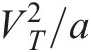that would make it drift toward the Equator were it not for the oblate geometry of the Earth (Figure 9). The resultant force of gravity (oriented toward the center of the Earth) and reaction (oriented normal to the surface) exactly cancels the centrifugal force, as shown in Figure 9. This should not be surprising considering that the oblate geometry is actually a consequence of the centrifugal force applied to the solid Earth. Consider now an eastward motion applied to the air parcel so that V > VT. This motion increases the centrifugal force and deflects the air parcel equatorward. Conversely, a westward motion with V < VT weakens the centrifugal force and deflects the air parcel poleward. In both cases the deflection is to the right in the northern hemisphere and to the left in the southern hemisphere.

Figure 9 Equilibrium of forces for an air parcel at rest in the frame of reference of the rotating Earth. The centrifugal force is directed away from the axis of rotation, gravity is directed toward the center of the Earth, and the reaction force is perpendicular to the surface. The centrifugal force would cause the air parcel to drift toward the Equator if the Earth were a perfect sphere. The oblate geometry of the Earth (greatly exaggerated for the purpose of this figure) results in equilibrium in the triangle of forces.Reproduced from Jacob. In summary, for an observer on the rotating Earth, air parcels moving horizontally are subject to a Coriolis force that is perpendicular to the direction of motion and proportional to the parcel’s velocity; this force deflects air parcels to the right in the northern hemisphere and to the left in the southern hemisphere. It can be shown that the corresponding Coriolis acceleration is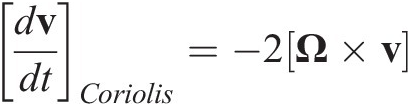(30)

where v represents the velocity vector in the rotating frame of reference and Ω is the Earth angular velocity vector directed from the south to the north pole. When expressed in Cartesian coordinates and considering the zonal and meridional wind components (u, v), the Coriolis acceleration becomes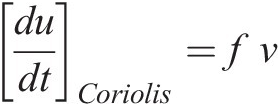(31)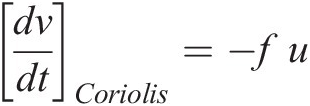(32)

where f = 2 Ω sin φ is the Coriolis parameter. It is positive in the northern hemisphere and negative in the southern hemisphere. Its amplitude increases with latitude. Thus, the Coriolis acceleration, which is zero at the Equator, increases with latitude and with the velocity of the flow. Its effect is substantial for large-scale motions (~1000 km, the synoptic scale).For an observer attached to the rotating Earth, the large-scale motions in the extratropical atmosphere can be represented by a balance between the Coriolis and the pressure-gradient forces, called the geostrophic approximation: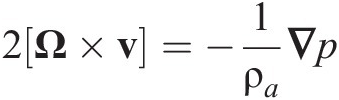(33)

or in a Cartesian projection (x and y being the geometric distances in the zonal and meridional directions, respectively)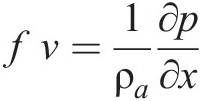(34)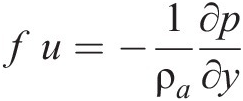(35)

From (33) we see that the geostrophic motions on a horizontal surface are parallel to the isobars (lines of constant pressure). In the northern hemisphere (f > 0), air parcels rotate clockwise around high pressure (anti-cyclonic) cells, and counter-clockwise around low pressure (cyclonic) cells (see Fig 10). The situation is reversed in the southern hemisphere (f < 0).

Fig 2.10 Flow of air in the northern hemisphere between anti-cyclonic (high) and cyclonic (low) regions. The motion originally directed from the high- to low-pressure cells is deflected to the right by the Coriolis force.When formulated using pressure rather than geometric altitude as the vertical coordinate, the geostrophic balance takes the form: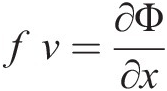(36)(37)

where Φ is the geopotential. Thus, on isobaric surfaces, the geostrophic motions follow the contours of the geopotential fields. Replacing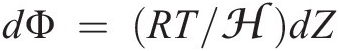yields the thermal wind equations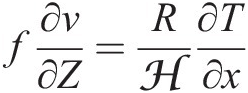(38)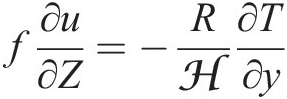(39)

These show that the vertical shear in the horizontal (constant pressure level) wind field is proportional to the horizontal temperature gradient. In both hemispheres, the zonal wind component u increases with height when temperature decreases with latitude and decreases with height when temperature increases with latitude (Fig 11). The strong decrease of temperature with latitude in the troposphere produces intense subtropical jet streams, seen in Fig 11 as westerly wind maxima centered at about 40° latitude and 10 km altitude.

Fig 11 Zonal mean temperature (a) and zonal wind velocity (b) as a function of latitude and altitude for January, from the COSPAR International Reference Atmosphere (CIRA).Reprinted with permission from Shepherd.

Near the surface, the geostrophic flow is modified by friction resulting from the loss of momentum as the flow encounters obstacles (vegetation, ocean waves, buildings, etc.). The friction force is directed in the direction opposite to the flow (slowdown of the wind), effectively weakening the Coriolis force. This deflects the flow toward areas of low pressure (or low geopotential areas on isobaric surfaces), as shown in Fig 12.

Figure 12 Winds around low- and high-pressure cells in the northern hemisphere. Geostrophic balance dominates aloft and the flow is directed along isobaric lines. Near the surface, friction deflects the flow toward low pressure.